# What are Broensted Lowry Acids

In 1923 independently prevailed Lowry in Cambridge and Br nsted (German spelling Br nsted) introduced the modern development of the acid / base term in Copenhagen. Initially, the term dealt exclusively with the water system. Br nsted But then extended it to acid / base reactions in non-aqueous solvents.

Proton donors and acceptors
To Br nsted are acidic compounds that can donate protons (Proton donors), and bases compounds that can accept protons (Proton acceptors). The definition of acids and bases can thus be reduced to the following equilibrium:Take the example of acetic acid (CH3COOH) in water (H.2O).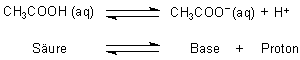But since a proton is extremely reactive, it is not so "naked", but binds to the water molecule: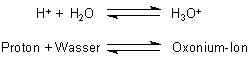(This is also an example of the Br nsted acid / base theory!)

Thus the whole reaction equation must be called as follows:In this example, the acetic acid releases a proton to the water, which in this case functions as a base and thus absorbs a proton and becomes the oxonium ion (H.3O+) becomes.

Once acid - always acid?
An equilibrium is established in this reaction. So we actually have two acids and two bases: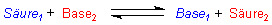If we stay in the example of dissolving acetic acid in water, the reaction looks like this: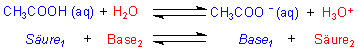During the forward reaction, the acid acetic acid releases a proton to the base water. This causes the acetate ion (CH3COO-) to the base and the water to the oxonium ion or the acid. In the reverse reaction, the oxonium ion releases a proton to the acetate ion, which in turn creates acetic acid and water.

Couples like CH3COOH and CH3COO- as well as H2O and H3O+ are each as conjugated acid / base pair because they belong together through the uptake or loss of a proton. The molecules with the same number (same color) in the reaction equation thus each represent such a corresponding acid / base pair.

In other words, one can also say:

H2O is the conjugate base to H3O+
H3O+ is the conjugated acid for H2O

CH3COO- is the conjugate base to CH3COOH
CH3COOH is the conjugated acid to CH3COO-

The stronger an acid, the weaker its corresponding base. Then there is no tendency for the acid to regress. One example is hydrochloric acid. Their base is the chloride ion. This is so weak that we don't have an acid / base equilibrium here; d. This means that the acid HCl is and remains completely dissociated.

HCl + H2O ———> Cl- + H3O+

Acid or base?
There are also many other molecules and ions that can occur both as bases and as acids.

In sulfuric acid we have two dissociation stages, which are characterized by a strong and a weak acid: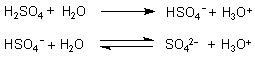We see that the HSO4-Ion is both a base (in the first reaction) and acid (in the second reaction). Such a substance is called an ampholyte; its property is called amphoteric.

Water is also an ampholyte. In the reaction with acetic acid it is a base (see above), whereas in the reaction with ammonia it occurs as an acid: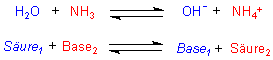The Br nsted acid / base theory has proven itself particularly with the ammonia system. This also applies to ammonia as an acid: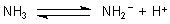The anion is a remarkably strong base; it is called amide ion.

The following table summarizes examples of amphoteric substances:

 Corresponding acid / base pairs Ampholyte Acid base H2O H2OH3O+ OH-H2O NH3 NH3NH4+ NH2-NH3 HSO4- HSO4-H2SO4 SO42-HSO4- HPO42- HPO42-H2PO4- PO43-HPO42-

Further texts on the subject of ammonia, amines and acid amides